Publication date: 07/30/2020

## Canonical Details

#### Details for the Test Details Option

When you select the Test Details option for a given test, eigenvalues, canonical correlations, and eigenvectors are shown in the report.

The canonical correlations produced by the Test Details option are computed as follows: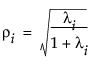where λi is the ith eigenvalue of the E-1H matrix used in computing the multivariate test statistics

The matrix labeled Eigvec is the V matrix, which is the matrix of eigenvectors of E-1H for the given test.

Note: The E and H matrices for the given test refer to M‘EM and M‘HM in terms of the original E and H matrices. The M matrix is defined by the response design. The E and H used in this section are defined in Multivariate Tests.

#### Details for Centroid Plot Option

The total sample centroid and centroid values for effects are computed as follows:

Grand =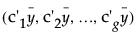Effectj =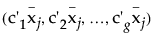where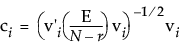N is the number of observations

vi is the ith column of V, the eigenvector matrix of E-1H for the given test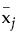is the multivariate least squares mean for the jth effect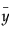is the overall mean of the responses

g is the number of eigenvalues of E-1H greater than 0

r is the rank of the X matrix

Note: The E and H matrices for the given test refer to M‘EM and M‘HM in terms of the original E and H matrices. The M matrix is defined by the response design. The E and H used in this section are defined in Multivariate Tests.

The centroid radii for effects are calculated as follows: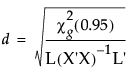where g is the number of eigenvalues of E-1H greater than 0 and the L matrices in the denominator are from the multivariate least squares means calculations.

#### Details for the Save Canonical Scores Option

The canonical Y values are calculated as follows: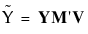where

Y is the matrix of response variables

M’ is the transpose of the response design matrix

V is the matrix of eigenvectors of E-1H for the given test

Note: The E and H matrices for the given test refer to M‘EM and M‘HM in terms of the original E and H matrices. The M matrix is defined by the response design. The E and H used in this section are defined in Multivariate Tests.

Canonical Y values are saved for eigenvectors corresponding to eigenvalues larger than zero.